# Difference between revisions of "12-240/Classnotes for Tuesday October 09"

In this lecture, the professor concentrated on bases and related theorems.

## Definition of basis

β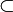$\subset \!\,$ V is a basis if

1/ It generates (span) V, span β = V

2/ It is linearly independent

## theorems

1/ β is a basis of V iff every element of V can be written as a linear combination of elements of β in a unique way.

proof: ( in the case β is finite)

β = {u1, u2, ..., un}

(<=) need to show that β = span(V) and β is linearly independent.

The fact that β span is the fact that every element of V can be written as a linear combination of elements of β, which is given

Assume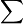$\sum \!\,$ ai∙ui = 0 ai$\in\!\,$ F, ui$\in\!\,$ β$\sum \!\,$ ai∙ui = 0 =$\sum \!\,$ 0.ui

since 0 can be written as a linear combination of elements of β in a unique way, ai=0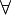$\forall\!\,$ i

Hence β is linearly independent

(=>) every element of V can be written as a linear combination of elements of β in a unique way.

So, suppose$\sum \!\,$ ai∙ui = v =$\sum \!\,$ bi∙ui

Thus$\sum \!\,$ ai∙ui -$\sum \!\,$ bi∙ui = 0$\sum \!\,$ (ai-bi)∙ui = 0

β is linear independent hence (ai - bi)= 0$\forall\!\,$ i

i.e ai = bi, hence the combination is unique.

## Clarification on lecture notes

On page 3, we find that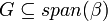$G \subseteq span(\beta)$ then we say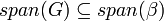$span(G) \subseteq span(\beta)$. The reason is, the Theorem 1.5 in the textbook.

Theorem 1.5: The span of any subset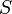$S$ of a vector space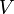$V$ is a subspace of$V$. Moreover, any subspace of$V$ that contains$S$ must also contain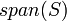$span(S)$

Since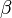$\beta$ is a subset of$V$,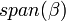$span(\beta)$ is a subspace of$V$ from the first part of the Theorem 1.5. We have shown (in the lecture notes page 3) that$G \subseteq span(\beta)$. From the "Moreover" part of Theorem 1.5, since$span(\beta)$ is a subspace of$V$ containing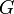$G$,$span(\beta)$ must also contain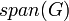$span(G)$.